# Money vs. Time-Weighted Return

Which does a better job of measuring an investment manager's performance?

## What are Money and Time-Weighted Returns?

Money and time-weighted returns are rates of return typically used to assess the performance of a managed investment portfolio. Today, the time-weighted rate of return is the industry standard since it provides a fairer assessment of an investment manager’s performance.### Money-Weighted Return

When it comes to monitoring investment performance, money-weighted returns function in the same way that internal rate of return (IRR) does. In a project assessment context, IRR would describe the return on investment of pursuing a particular project. The cost of capital (WACC) can be thought of as the minimum benchmark IRR that a project must produce in order to have a positive NPV. From a mathematical standpoint:

• WACC > IRR = Negative NPV
• WACC = IRR = NPV of \$0
• WACC < IRR = Positive NPV

In an investment management context, the money-weighted return is the return at which a portfolio’s present value (PV) of inflows equals its PV of outflows (i.e., the rate of return that equals the discount rate). Examples of cash inflows and outflows can be found below: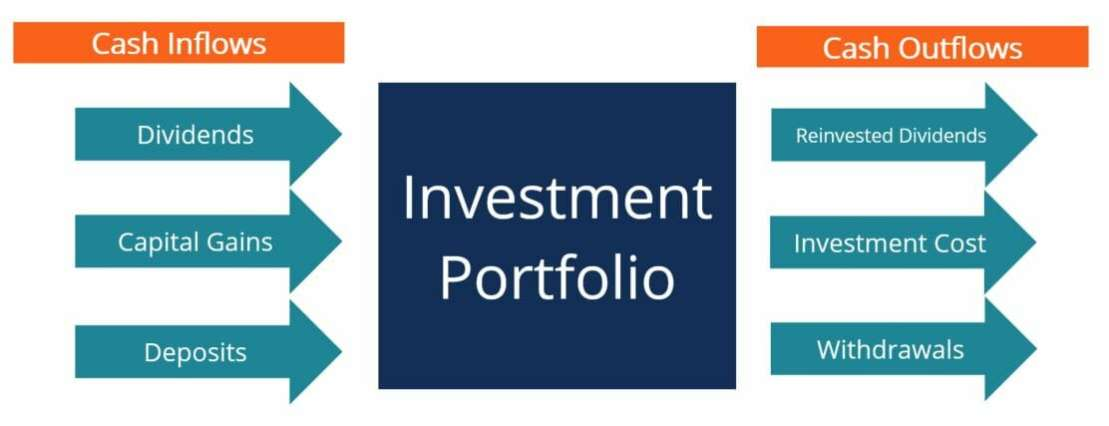This method is not ideal to monitor the performance of investment managers, since returns can be skewed by large deposits or withdrawals requested by clients. Other such changes to account size may unfairly help or penalize an investment manager since client requests are out of their control.

To calculate money-weighted return, set the PV of cash inflows = PV cash outflows and solve for the discount rate. This will require a spreadsheet or a financial calculator. To compute the money-weighted return, we will need to:

1. Identity all outflows and inflows
2. Set PV outflows = PV inflows
3. Solve for r

#### Money-Weighted Return Example

An investment manager purchases a stock today for \$100. He intends to hold the stock for three years and collects \$5 in dividends each year. At the end of the third year, he expects to be able to sell the stock for \$150. What is the money-weighted return on this investment portfolio?

Step 1 – Identify inflows and outflows

Inflows: Dividends (\$5 in Years 1,2 and 3) and Sale of stock (\$150 in Year 3). Outflows: Purchase of stock (Year 0)

Step 2 – Set PV inflows = PV outflows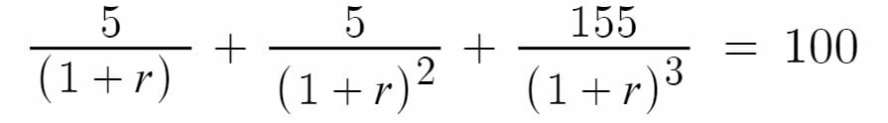Step 3 – Solve for r

Using a financial calculator, solver on excel or through trial and error, we arrive at a value of approximately 18.88%

### Time-Weighted Return

Time-weighted returns are geometric means of the performance of investment portfolios. Calculating time-weighted return requires breaking up an investment portfolio across various time intervals (or holding intervals) and evaluating performance during each interval (thus the name “time-weighted”). Portfolio performance for each holding interval can be found using the following equation: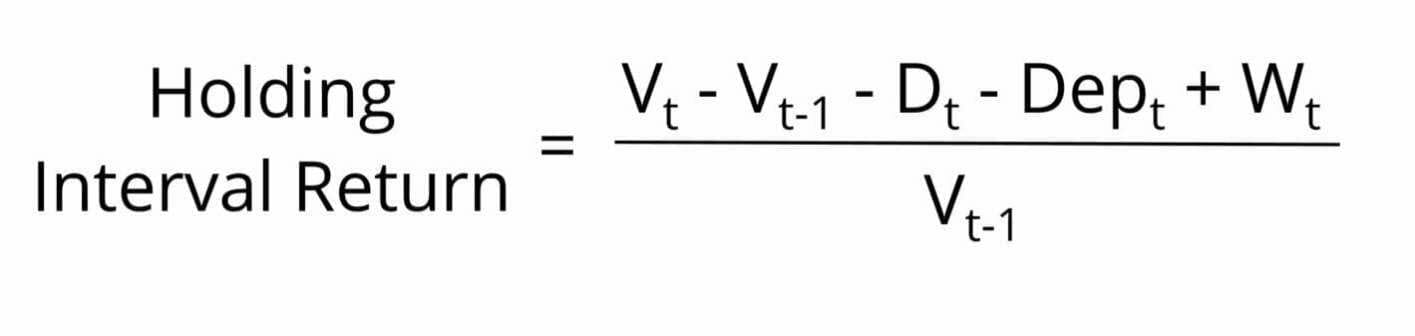Where:

Vt – Value of portfolio at the end of period t

Vt-1 – Value of portfolio at end of period t-1 (the previous period)

Dt – Dividends received during period t

Dept – Deposits made during period t

Wt – Withdrawals made during period t

This approach isolates investment performance from withdrawals and deposits, making the assessment of the investment manager fairer. Withdrawals and deposits will be accounted for in the following period’s Vt.

To calculate the time-weighted return of an investment portfolio, we must:

1. Identify all holding intervals
2. Identify all withdrawals and deposits
3. Calculate holding interval return (HIR) for each interval
4. Find the geometric mean of the HIRs by adding 1 to each return and multiplying them together, then subtracting 1
5. Annualize return

#### Time-Weighted Return Example

The table below describes the market value of a certain portfolio at different dates: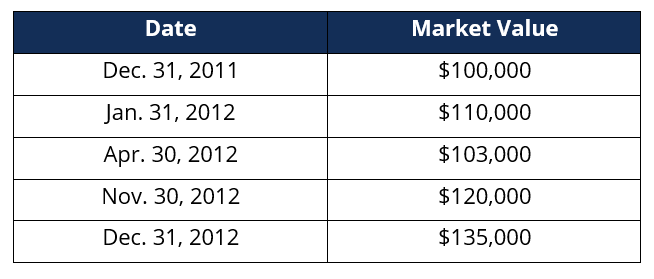We also learn that the client made a \$12,000 withdrawal on March 3, 2012 and a \$20,000 deposit on December 20, 2012. What is the time-weighted return of this investment portfolio?

Step 1 – Identify all holding intervals

Based on the given information, there are 4 holding intervals (HIs):

• From Dec. 31, 2011 to Jan. 31 2012 (HI1)
• From Jan. 31 2012 to Apr. 30 2012 (HI2)
• From Apr. 30 2012 to Nov. 30 2012 (HI3)
• From Nov 30. 2012 to Dec 31. 2012 (HI4)

Step 2 – Identify all withdrawals and deposits

• There is a \$12,000 withdrawal made on March 3, 2012, which falls in HI2
• There is a \$20,000 deposit made on December 20, 2012, which falls in HI4

Step 3 – Calculate holding interval return (HIR) for each interval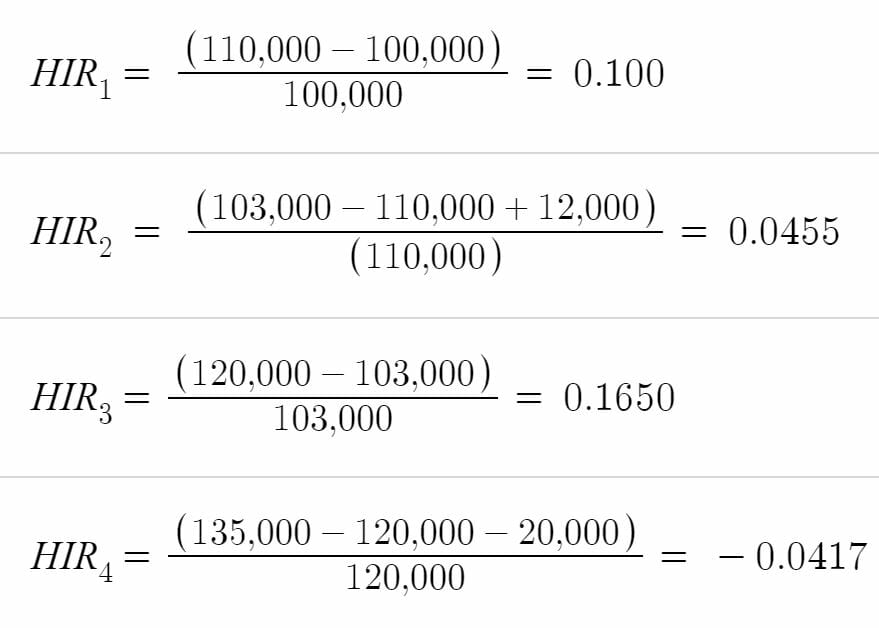Step 4 – Add 1 to HIRs, multiplying them together, then subtracting 1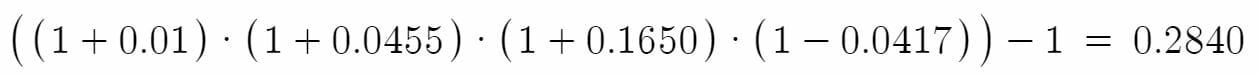Step 5 – Annualize returns

The data provided above is for one year, thus, there is no need to conduct the annualization calculation. Had the data provided covered more than one year, we would need to follow this formula.

Thus, the time-weighted return on this portfolio is of 28.40%

### Conclusion

Both methods are useful in evaluating the performance of an investment portfolio over time. However, as described above, the time-weighted method is a more practical formula since it factors out changes in account balances and focuses solely on investment performance.

### More Resources

Thank you for reading CFI’s guide to money vs. time-weighted return. CFI offers the Financial Modeling & Valuation Analyst (FMVA)™ certification program for those looking to take their careers to the next level. To learn more about related topics, check out the following resources:

• Investment Methods
• Total Return Swap
• Rate of Return
• Return on Assets (ROA)
• Fixed Income Fundamentals Course – CFI

### Financial Analyst Training

Get world-class financial training with CFI’s online certified financial analyst training program!

Gain the confidence you need to move up the ladder in a high powered corporate finance career path.

Learn financial modeling and valuation in Excel the easy way, with step-by-step training.